# Wielandt subgroup

(diff) ← Older revision | Latest revision (diff) | Newer revision → (diff)

The Wielandt subgroup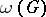of a groupis defined to be the intersection of the normalizers of all the subnormal subgroups of(cf. also Subnormal subgroup; Normal subgroup; Normalizer of a subset). This characteristic subgroup was introduced in 1958 by H. Wielandt [a18]. Note that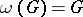if and only ifis a T-group, i.e. normality is transitive in.

Whenis nilpotent (cf. Nilpotent group),is the intersection of the normalizers of all the subgroups of. The latter is called the norm ofand was introduced by R. Baer [a1]. In [a17], E. Schenkman showed that the norm is always contained in the second centre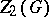(cf. also Centre of a group). Hence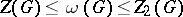ifis a nilpotent group.

The upper Wielandt series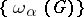of a groupis formed by iteration; thus,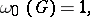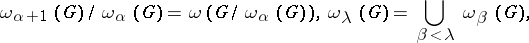whereis an ordinal anda limit ordinal (cf. also Ordinal number). Iffor some ordinal, the smallest suchis called the Wielandt length of. Ifhas finite Wielandt length, then every subnormal subgroup ofhas defect at most.

Wielandt [a18] proved thatcontains all the subnormal (non-Abelian) simple subgroups of, and also all the minimal normal subgroups ofthat satisfy min-sn, the minimal condition on subnormal subgroups (cf. also Group with the minimum condition). This last result implies thatwheneveris a non-trivial group with min-sn. Thus, the Wielandt subgroup is unexpectedly large for groups with min-sn.

A stronger result in this direction was found independently by D.J.S. Robinson [a15] and J.E. Roseblade [a16]: Ifis a group with min-sn, then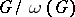is finite. Thus, a subnormal subgroup ofhas only finitely many conjugates. In addition, O.H. Kegel [a11] generalized the first of Wielandt's results by demonstrating thatcontains all subnormal perfect T-subgroups of of. Since solvable T-groups are metAbelian ([a14]),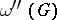is a perfect T-group. It follows that the join (cf. also Join) of all the subnormal perfect T-subgroups coincides within any group. For a smooth treatment of these results see [a12].

The example of the infinite dihedral group shows that the Wielandt subgroup of a polycyclic group can easily be trivial. In 1991, J. Cossey [a7] showed that ifis a polycyclic group, thenis finite. Cossey also proved that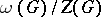is finite ifis nilpotent-by-Abelian. Subsequently, this conclusion was extended to polycyclic groups that are meta-nilpotent or Abelian-by-finite by R. Brandl, S. Franciosi and F. de Giovanni. These authors were also able to show that ifis a finitely generated solvable-by-finite group of finite Prüfer rank (cf. Rank), thenis contained in the FC-centre (cf. also Group with a finiteness condition).

In some waysmight seem to be close to the centre for polycyclic groups. However, Cossey [a7] has pointed out that there is a nilpotent-by-finite polycyclic groupsuch thatis non-trivial and free Abelian. Cossey [a7] has also investigated the Wielandt length of a polycyclic group, showing that this exists if and only ifis finite-by-nilpotent (when, of course, the Wielandt length is finite).

The Wielandt length of finite solvable groups has been investigated extensively by several authors, and its connection with other invariants such as the derived length and nilpotent length has been analyzed. Letbe a finite solvable group with Wielandt length. Since solvable T-groups are metAbelian, the derived lengthofis at most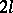. A.R. Camina [a5] improved this bound by showing that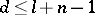, whereis the nilpotent length ofand. Camina also showed that. Subsequently, R. Bryce and J. Cossey [a4] gave the improved estimate, which is best possible when. This work has been extended by C. Casolo [a6] to infinite solvable groups with finite Wielandt length. For more results on the Wielandt length of finite groups, see [a8].

In [a4], a local version of the Wielandt subgroup was introduced,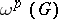whereis a prime number; this is the intersection of the normalizers of the-perfect subnormal subgroups, i.e. those that have no non-trivial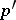-quotients. They showed that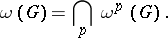Another variation of the Wielandt subgroup has been described in [a2]. The generalized Wielandt subgroup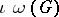of a groupis defined to be the intersection of the normalizers of all the infinite subnormal subgroups of. Thus,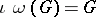precisely whenis an IT-group, i.e. all infinite subnormal subgroups ofare normal. The class of IT-groups has been investigated in [a10] and [a9]. J.C. Beidleman, M.R. Dixon and D.J.S. Robinson [a2] showed that the subgroupsandare remarkably close, and indeed they coincide in many cases. In [a2] the following results were established. Letbe an infinite group; then: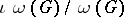is a residually finite T-group (cf. also Residually-finite group);unless the subgroupgenerated by all the finite solvable subnormal subgroups ofis Prüfer-by-finite;

ifis Prüfer-by-finite and infinite, thenis metAbelian;

ifis finite, so is. Finally,ifis a finitely generated infinite solvable group.

How to Cite This Entry:
Wielandt subgroup. Encyclopedia of Mathematics. URL: http://encyclopediaofmath.org/index.php?title=Wielandt_subgroup&oldid=18195
This article was adapted from an original article by Derek J.S. Robinson (originator), which appeared in Encyclopedia of Mathematics - ISBN 1402006098. See original article